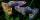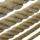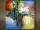# Ages

John, Teresa, Daniel and Paul have summary 56 years. Their ages are in a ratio of 1:2:5:6. Determine how many years have each of them.

Result

j =  4
t =  8
d =  20
p =  24

#### Solution:Leave us a comment of example and its solution (i.e. if it is still somewhat unclear...):

Showing 0 comments:Be the first to comment!## Next similar examples:

1. Moneys in triple ratioMilan, John and Lili have a total 344 euros. Their amounts are in the ratio 1:2:5. Determine how much each of them has?
2. GuppiesAudrey has some guppies in a fish tank. The ration of the oranges guppies to silver guppies is 3:5. She has 12y oranges guppies. Write the number of silver guppies she has in terms of y
3. PeppersIn the box are yellow (a), green (b) and red (c) peppers. Their amount is in a ratio 2:4:1 . Most are yellow peppers and green the least. Calculate the number of peppers each type if the total number of peppers is 70.
4. DonutsFind how many donuts each student will receive if you share 126 donuts in a ratio of 1:5:8
5. RunnersFor three runners (on the first to third place) is prepared 30 chocolate that they be distributed in the ratio of 3 : 2 : 1 How much chocolate will get everyone?
6. StringDivide the string length 800 cm in ratio 1: 5
7. Line segmentThe 4 cm long line segment is enlarged in the ratio of 5/2. How many centimeters will measure the new line segment?
8. BuildingAt the building, we divided 240 boards into two piles in a 5: 3 ratio. How many were fewer boards in the lower pile?
9. RatiosReduce the numbers: 50 in a 1:2 ratio 111 at a ratio of 2:3 70 at 10:50 560 at a ratio of 3:8
10. Land areaA land area of Asia and Africa are in a 3: 2 ratio, the European and African are is 1:3. What are the proportions of Asia, Africa, and Europe?
11. Divide moneyDivide 1200 USD at a ratio of 1:2:3:4:5:6:9:10
12. Image scaleThe actual image dimensions are 60 cm x 80cm and has a reduced size 3 cm x 4 cm. At what scale the image was reduced?
13. One-thirdA one-third of unknown number is equal to five times as great as the difference of the same unknown number and number 28. Determine the unknown number.
14. QuizTested student answered correctly on a 4/ 8 questions. Wrong answers was 16. How many questions answered?
15. 1.5 divided1.5 divided by 1 = w divided by 4
16. Masons1 mason casts 30.8 meters square in 8 hours. How long casts 4 masons 178 meters square?
17. Equation?